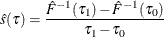# The HPQUANTSELECT Procedure

#### Linear Model with iid Errors

You can specify the SPARSITY(IID) option in the MODEL statement to assume that the distribution of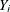conditional on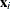follows the linear model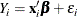where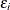for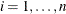are iid in the distribution function F. Let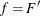denote the density function of F. Further assume that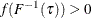in a neighborhood of. Then, under some mild conditions, Koenker and Bassett (1982) prove that the asymptotic distribution of the quantile regression estimates is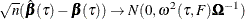where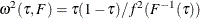and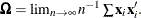The reciprocal of the density function,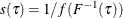, is called the sparsity function.

Accordingly, the covariance matrix of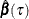can be estimated as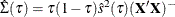where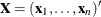is the design matrix and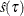is an estimate of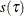. Under the iid assumption, the algorithm for computingis as follows:

1. Fit a quantile regression model and compute the residuals. Each residual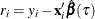can be viewed as an estimated realization of the corresponding error.

2. Compute the quantile level bandwidth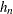. The HPQUANTSELECT procedure provides two bandwidth methods:

• The Bofinger bandwidth is an optimizer of mean squared error for standard density estimation: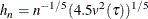• The Hall-Sheather bandwidth is based on Edgeworth expansions for studentized quantiles,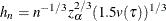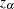satisfies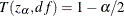for the construction of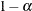confidence intervals, where T is the cumulative distribution function for the t distribution and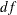is the residual degrees of freedom.

The quantity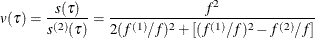is not sensitive to f and can be estimated by assuming f is Gaussian as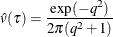where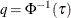.

3. Compute residual quantiles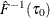and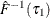as follows:

1. Set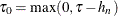and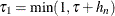.

2. Use the equation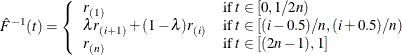where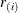is the ith smallest residual and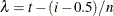.

3. If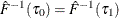, find i that satisfies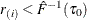and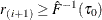. If such an i exists, reset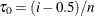so that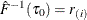. Also find j that satisfies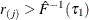and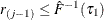. If such a j exists, reset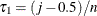so that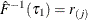.

4. Estimate the sparsity functionas